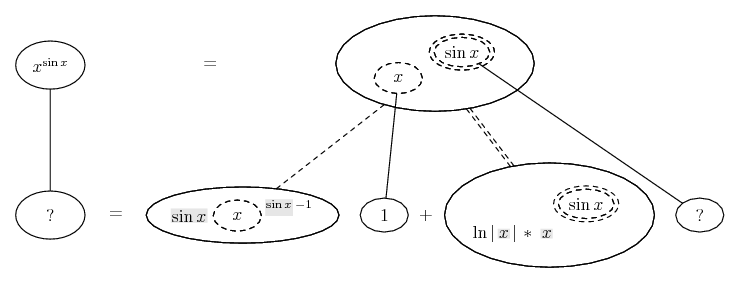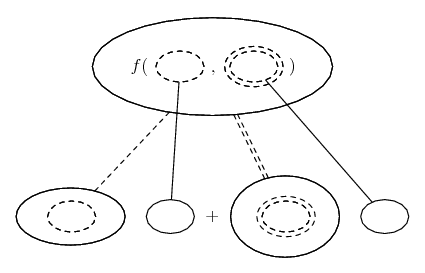# Thread: Derivative

1. ## Derivative

Can someone please explain how to take the derivative of x^sin(x)

Thanks...

2. Generally speaking the simplest way to differentiate functions in which the variable, x, appears in both base and exponent is to take the logarithm of the function first. If $\displaystyle y= x^{sin(x)}$ then $\displaystyle ln(y)= ln(x^{sin(x)})= sin(x) ln(x)$. Use the product rule on the right and don't forget that, by the chain rule on the left, the derivative of ln(y) is (1/y) y'. Solve the equation for y'.

3. The derivative of ln(y) is just 1/y right?

So i have: 1/y = cos(x)*ln(x) + sin(x)*(1/x) after the product rule

Now just solve for y?

So the answer is: 1 / cos(x)*ln(x) + sin(x)*(1/x)

4.Originally Posted by jzelltThe derivative of ln(y) is just 1/y right? Mr F says: No. See below.

So i have: 1/y = cos(x)*ln(x) + sin(x)*(1/x) after the product rule

Now just solve for y?

So the answer is: 1 / cos(x)*ln(x) + sin(x)*(1/x)
$\displaystyle \frac{d (\ln y)}{dx} = \frac{d (\ln y)}{dy} \cdot \frac{dy}{dx} = \frac{1}{y} \frac{dy}{dx}$ so you make the derivative the subject by multiplying both sides of the equation by y and then replace y with $\displaystyle x^{\sin x}$.

5.Originally Posted by jzelltThe derivative of ln(y) is just 1/y right?
I said in my first response, "don't forget that, by the chain rule on the left, the derivative of ln(y) is (1/y) y'."

So i have: 1/y = cos(x)*ln(x) + sin(x)*(1/x) after the product rule
Now just solve for y?
And I said "Solve the equation for y'."

So the answer is: 1 / cos(x)*ln(x) + sin(x)*(1/x)
No, it isn't. Go back and do what I told you to do,

6. Just in case a choice of methods is helpful (not always, so do ignore if you want)...... where... is the chain rule for two inner functions, i.e...

$\displaystyle \frac{d}{dx}\ f(u(x), v(x)) = \frac{\partial f}{\partial u} \frac{du}{dx} + \frac{\partial f}{\partial v} \frac{dv}{dx}$

... which is obviously best avoided at this level, but notice that Wolfram will choose this method for its 'show steps', and I hope the picture is a bit easier to follow - or at least enlightening to peruse. As with...... the ordinary chain rule, straight continuous lines differentiate downwards (integrate up) with respect to x, and the straight dashed line similarly but with respect to the (corresponding) dashed balloon expression which is (one of) the inner function(s) of the composite expression.

When differentiating with respect to, say the single dashed balloon, you treat the other as a constant - expressions are shaded in the picture to show they've been treated as constants on the way down (during differentiation). I've left a couple of blanks to fill. You might want to compare also with http://www.mathhelpforum.com/math-he...tml#post374503.

Finally, I must acknowledge that to see the logic of the double-dashed differentiation, i.e. to see why

$\displaystyle \frac{d}{du} a^u = \ln a\ a^u$

you do of course need logarithmic differentiation as explained by the others, above.

_____________________________________

Don't integrate - balloontegrate!

Balloon Calculus Forum

Balloon Calculus Drawing with LaTeX and Asymptote!

#### Search Tags

derivative